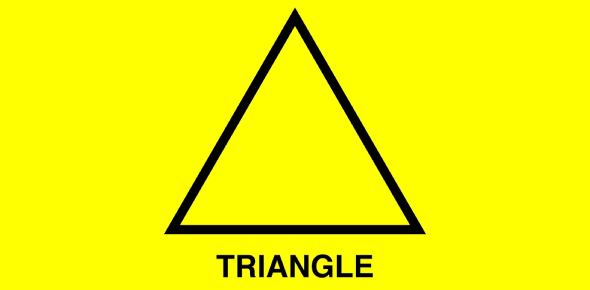# 5.1-5.3 Quiz Review

15 Questions | Total Attempts: 283SettingsThis is a review for the points of concurrency quiz that you will be taking in class shortly. Spelling counts.

• 1.
What is the point of concurrency of perpendicular bisectors of a triangle called?
• 2.
In a right triangle, the incenter is at what specific location?
• 3.
The circumcenter of a triangle is equidistant from the _____________ of the triangle.
• 4.
When the centroid of a triangle is constructed, it divides the median segments into parts that are proportional.  What is the fractional relationship between the smallest part of the median segment and the larger part of the median segment?
• 5.
The centroid of a triangle is (sometimes, always, or never) inside the triangle.
• 6.
The circumcenter of a triangle is the center of the circle that circumscribes the triangle, intersecting each _______ of the triangle.
• 7.
What is the point of concurrency of angle bisectors of a triangle called?
• 8.
What is the point of concurrency of the medians of a triangle called?
• 9.
What is the point of concurrency of the altitudes of a triangle called?
• 10.
The incenter of a triangle is the center of the circle that is inscribed inside the triangle, intersecting each ______ of the triangle.
• 11.
The circumcenter of a triangle is (sometimes, always or never) inside the triangle.
• 12.
The incenter of a triangle is equidistant from the ________ of the triangle.
• 13.
The incenter of a triangle is (sometimes, always, or never) inside the triangle.
• 14.
The orthocenter of a triangle is (sometimes, always, or never) inside the triangle.
• 15.
In a right triangle, the centroid is at what specific location?
Related Topics